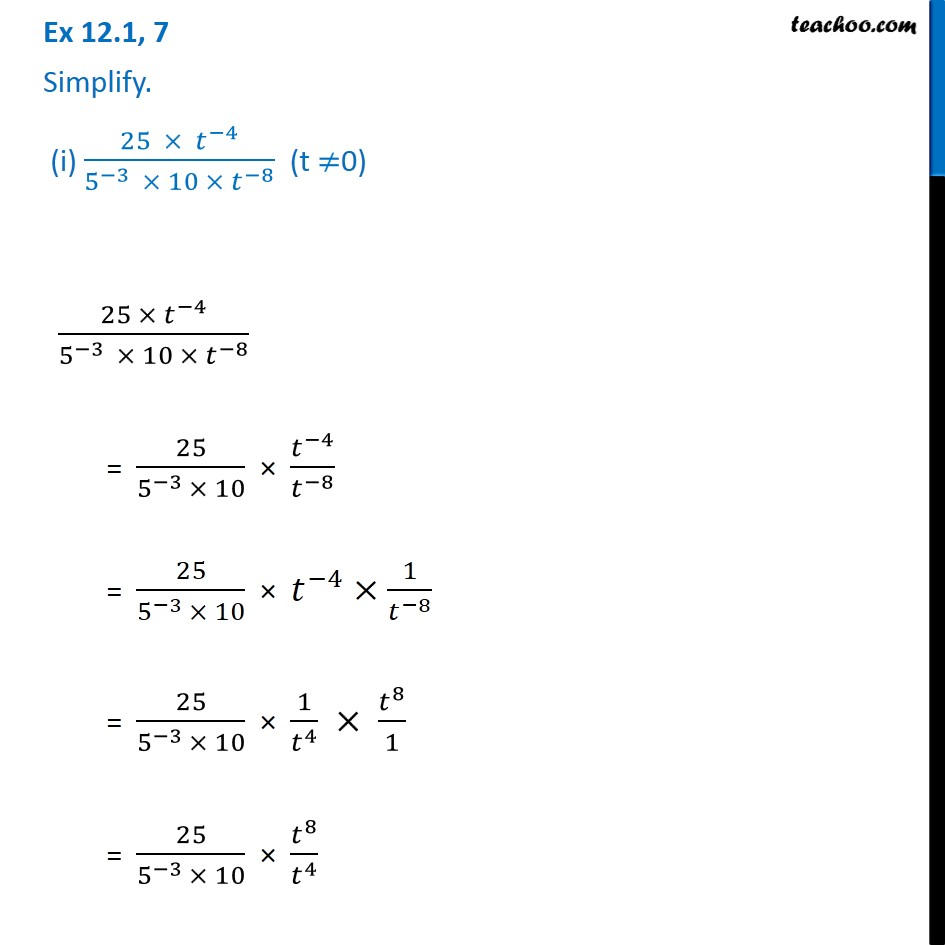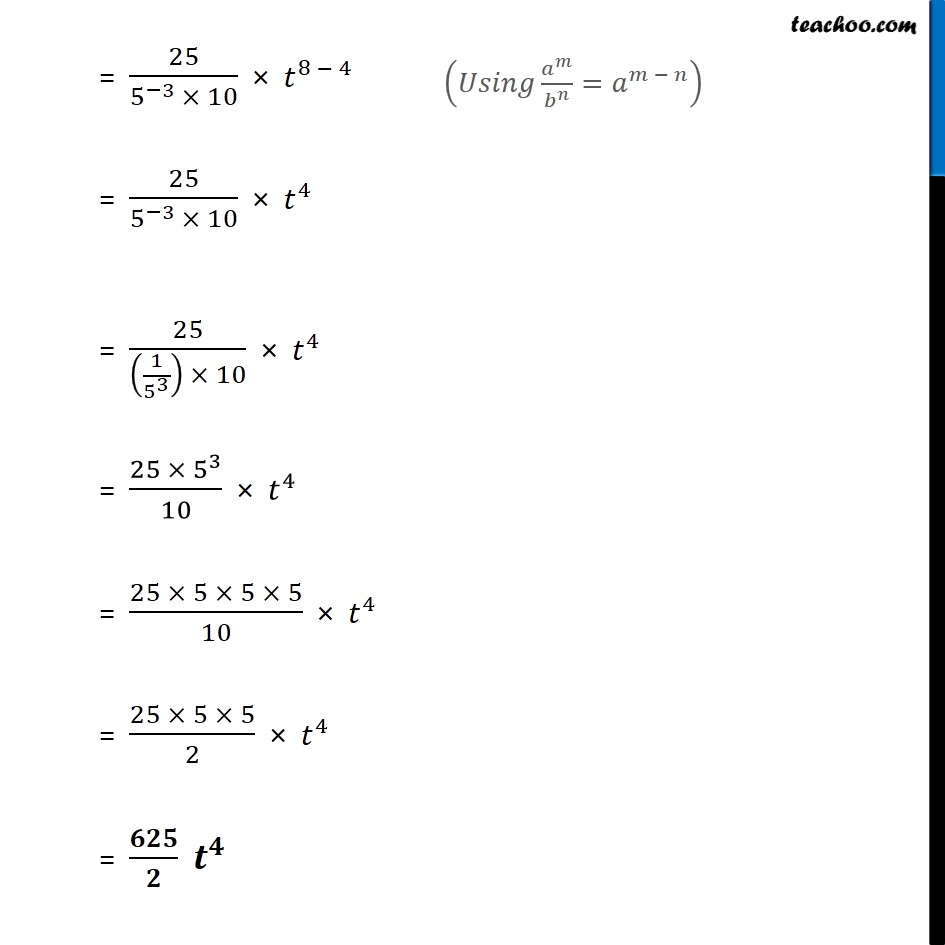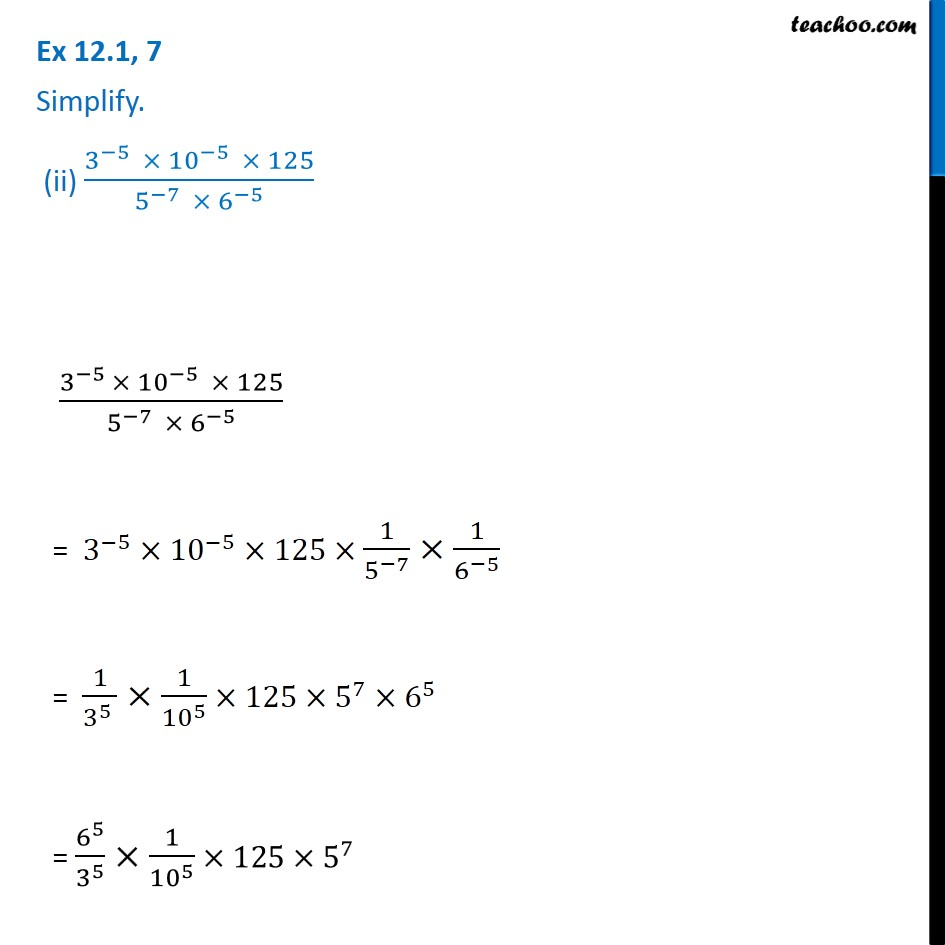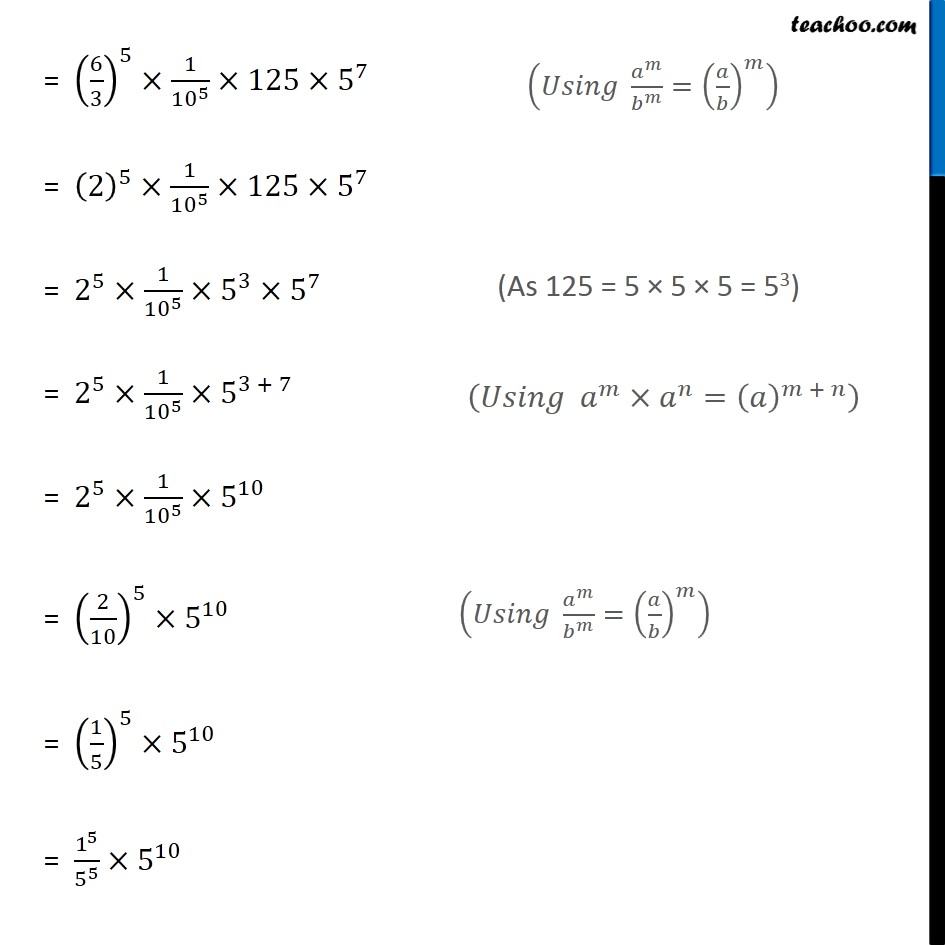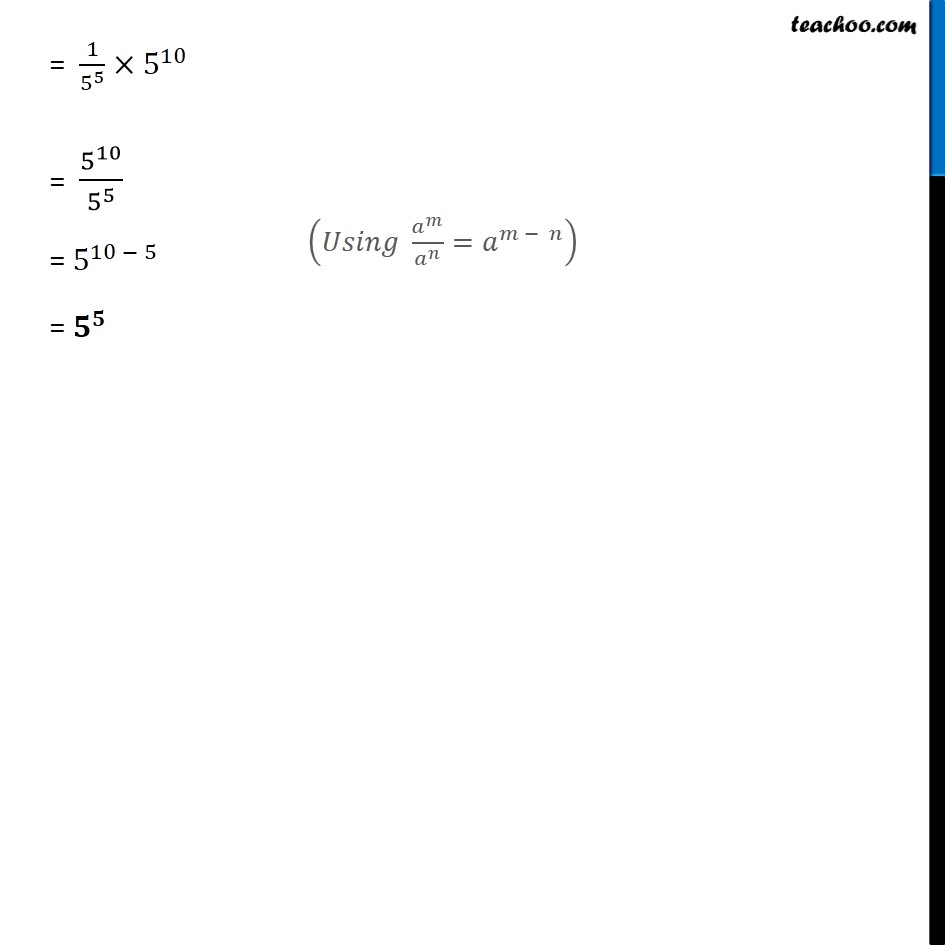1. Chapter 12 Class 8 Exponents and Powers
2. Serial order wise
3. Ex 12.1

Transcript

Ex 12.1, 7 Simplify. (i) (25 × 𝑡^(−4))/(5^(−3 ) × 10 × 𝑡^(−8) ) (t ≠0) (25 × 𝑡^(−4))/(5^(−3 ) × 10 × 𝑡^(−8) ) = 25/(5^(−3 )× 10) × 𝑡^(−4)/𝑡^(−8) = 25/(5^(−3 )× 10) × 𝑡^(−4)×1/𝑡^(−8) = 25/(5^(−3 )× 10) × 1/𝑡^4 × 𝑡^8/1 = 25/(5^(−3 )× 10) × 𝑡^8/𝑡^4 = 25/(5^(−3 )× 10) × 𝑡^(8 − 4) = 25/(5^(−3 )× 10) × 𝑡^4 = 25/((1/5^3 ) × 10) × 𝑡^4 = (25 × 5^3)/10 × 𝑡^4 = (25 × 5 × 5 × 5)/10 × 𝑡^4 = (25 × 5 × 5)/2 × 𝑡^4 = 𝟔𝟐𝟓/𝟐 𝒕^𝟒 (𝑈𝑠𝑖𝑛𝑔 𝑎^𝑚/𝑏^𝑛 =𝑎^(𝑚 − 𝑛) ) Ex 12.1, 7 Simplify. (ii) (3^(−5 ) × 〖10〗^(−5 ) × 125)/(5^(−7 ) × 6^(−5) ) (3^(−5 )× 〖10〗^(−5 ) × 125)/(5^(−7 ) × 6^(−5) ) = 3^(−5)×〖10〗^(−5)×125× 1/5^(−7) ×1/6^(−5) = 1/3^(5 ) ×1/〖10〗^5 ×125×5^7×6^5 = 6^5/3^5 ×1/〖10〗^5 ×125×5^7 = (6/3)^5×1/〖10〗^5 ×125×5^7 = (2)^5×1/〖10〗^5 ×125×5^7 = 2^5×1/〖10〗^5 ×5^3×5^7 = 2^5×1/〖10〗^5 ×5^(3 + 7) = 2^5×1/〖10〗^5 ×5^10 = (2/10)^5×5^10 = (1/5)^5×5^10 = 1^5/5^5 ×5^10 (𝑈𝑠𝑖𝑛𝑔 𝑎^𝑚/𝑏^𝑚 =(𝑎/𝑏)^𝑚 ) (As 125 = 5 × 5 × 5 = 53) (𝑈𝑠𝑖𝑛𝑔 𝑎^𝑚×𝑎^𝑛=(𝑎)^(𝑚 + 𝑛) ) (𝑈𝑠𝑖𝑛𝑔 𝑎^𝑚/𝑏^𝑚 =(𝑎/𝑏)^𝑚 ) = 1/5^5 ×5^10 = 5^10/5^5 = 5^(10 − 5) = 𝟓^𝟓 (𝑈𝑠𝑖𝑛𝑔 𝑎^𝑚/𝑎^𝑛 =𝑎^(𝑚 − 𝑛) )

Ex 12.1